# Title 40

## SECTION 1065.680

### 1065.680 Adjusting emission levels to account for infrequently regenerating aftertreatment devices.

§ 1065.680 Adjusting emission levels to account for infrequently regenerating aftertreatment devices.

This section describes how to calculate and apply emission adjustment factors for engines using aftertreatment technology with infrequent regeneration events that may occur during testing. These adjustment factors are typically calculated based on measurements conducted for the purposes of engine certification, and then used to adjust the results of testing related to demonstrating compliance with emission standards. For this section, “regeneration” means an intended event during which emission levels change while the system restores aftertreatment performance. For example, exhaust gas temperatures may increase temporarily to remove sulfur from adsorbers or to oxidize accumulated particulate matter in a trap. Also, “infrequent” refers to regeneration events that are expected to occur on average less than once over a transient or ramped-modal duty cycle, or on average less than once per mode in a discrete-mode test.

(a) Apply adjustment factors based on whether there is active regeneration during a test segment. The test segment may be a test interval or a full duty cycle, as described in paragraph (b) of this section. For engines subject to standards over more than one duty cycle, you must develop adjustment factors under this section for each separate duty cycle. You must be able to identify active regeneration in a way that is readily apparent during all testing. All adjustment factors for regeneration are additive.

(1) If active regeneration does not occur during a test segment, apply an upward adjustment factor, UAF, that will be added to the measured emission rate for that test segment. Use the following equation to calculate UAF: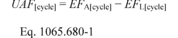Where: EFA[cycle] = the average emission factor over the test segment as determined in paragraph (a)(4) of this section. EFL[cycle] = measured emissions over a complete test segment in which active regeneration does not occur. Example: EFARMC = 0.15 g/kW·hr EFLRMC = 0.11 g/kW·hr UAFRMC = 0.15 − 0.11 = 0.04 g/kW·hr

(2) If active regeneration occurs or starts to occur during a test segment, apply a downward adjustment factor, DAF, that will be subtracted from the measured emission rate for that test segment. Use the following equation to calculate DAF: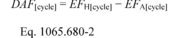Where: EFH[cycle] = measured emissions over the test segment from a complete regeneration event, or the average emission rate over multiple complete test segments with regeneration if the complete regeneration event lasts longer than one test segment. Example: EFARMC = 0.15 g/kW·hr EFHRMC = 0.50 g/kW·hr DAFRMC = 0.50 − 0.15 = 0.35 g/kW·hr

(3) Note that emissions for a given pollutant may be lower during regeneration, in which case EFL would be greater than EFH, and both UAF and DAF would be negative.

(4) Calculate the average emission factor, EFA, as follows: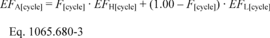Where: F[cycle] = the frequency of the regeneration event during the test segment, expressed in terms of the fraction of equivalent test segments during which active regeneration occurs, as described in paragraph (a)(5) of this section. Example: FRMC = 0.10 EFARMC = 0.10 · 0.50 + (1.00 − 0.10) · 0.11 = 0.15 g/kW·hr

(5) The frequency of regeneration, F, generally characterizes how often a regeneration event occurs within a series of test segments. Determine F using the following equation, subject to the provisions of paragraph (a)(6) of this section: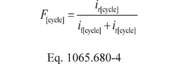Where: ir[cycle] = the number of successive test segments required to complete an active regeneration, rounded up to the next whole number. if[cycle] = the number of test segments from the end of one complete regeneration event to the start of the next active regeneration, without rounding. Example: irRMC = 2 ifRMC = 17.86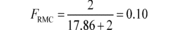(6) Use good engineering judgment to determine ir and if, as follows:

(i) For engines that are programmed to regenerate after a specific time interval, you may determine the duration of a regeneration event and the time between regeneration events based on the engine's design parameters. For other engines, determine these values based on measurements from in-use operation or from running repetitive duty cycles in a laboratory.

(ii) For engines subject to standards over multiple duty cycles, such as for transient and steady-state testing, apply this same calculation to determine a value of F for each duty cycle.

(iii) Consider an example for an engine that is designed to regenerate its PM filter 500 minutes after the end of the last regeneration event, with the regeneration event lasting 30 minutes. If the RMC takes 28 minutes, irRMC = 2 (30 ÷ 28 = 1.07, which rounds up to 2), and ifRMC = 500 ÷ 28 = 17.86.

(b) Develop adjustment factors for different types of testing as follows:

(1) Discrete-mode testing. Develop separate adjustment factors for each test mode (test interval) of a discrete-mode test. When measuring EFH, if a regeneration event has started but is not complete when you reach the end of the sampling time for a test interval, extend the sampling period for that test interval until the regeneration event is complete.

(2) Ramped-modal and transient testing. Develop a separate set of adjustment factors for an entire ramped-modal cycle or transient duty cycle. When measuring EFH, if a regeneration event has started but is not complete when you reach the end of the duty cycle, start the next repeat test as soon as possible, allowing for the time needed to complete emission measurement and installation of new filters for PM measurement; in that case EFH is the average emission level for the test segments that included regeneration.

(3) Accounting for cold-start measurements. For engines subject to cold-start testing requirements, incorporate cold-start operation into your analysis as follows:

(i) Determine the frequency of regeneration, F, in a way that incorporates the impact of cold-start operation in proportion to the cold-start weighting factor specified in the standard-setting part. You may use good engineering judgment to determine the effect of cold-start operation analytically.

(ii) Treat cold-start testing and hot-start testing together as a single test segment for adjusting measured emission results under this section. Apply the adjustment factor to the composite emission result.

(iii) You may apply the adjustment factor only to the hot-start test result if your aftertreatment technology does not regenerate during cold operation as represented by the cold-start transient duty cycle. If we ask for it, you must demonstrate this by engineering analysis or by test data.

(c) If an engine has multiple regeneration strategies, determine and apply adjustment factors under this section separately for each type of regeneration.

[81 FR 74189, Oct. 25, 2016]